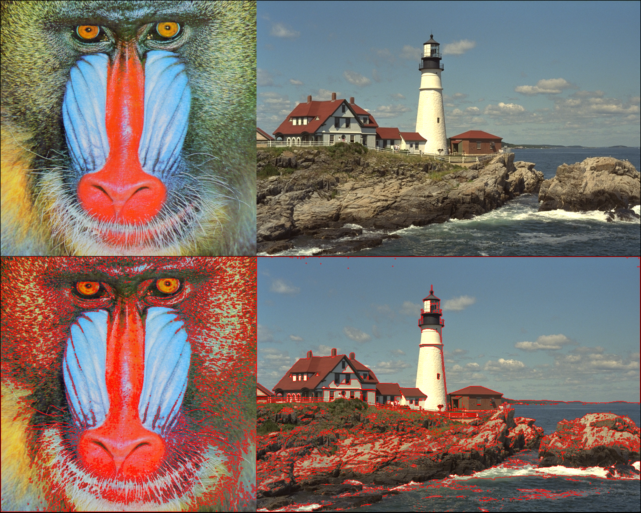# Contour Detection and DrawingThis demonstration shows how to detect contours on binary images The algorithm used is "Topological Structural Analysis of Digitized Binary Images by Border Following" by Suzuki and Abe (Same as OpenCV).#

Points are represented using CartesianIndex. Contours are represented as a vector of Points. Direction is a single number between 1 to 8. Steps inside functions are marked as they are in the original paper

using Images, TestImages, FileIO

#              N          NE      E       SE      S       SW        W      NW
# direction between two pixels

# rotate direction clockwise
function clockwise(dir)
return (dir)%8 + 1
end

# rotate direction counterclockwise
function counterclockwise(dir)
return (dir+6)%8 + 1
end

# move from current pixel to next in given direction
function move(pixel, image, dir, dir_delta)
newp = pixel + dir_delta[dir]
height, width = size(image)
if (0 < newp <= height) &&  (0 < newp <= width)
if image[newp]!=0
return newp
end
end
return CartesianIndex(0, 0)
end

# finds direction between two given pixels
function from_to(from, to, dir_delta)
delta = to-from
return findall(x->x == delta, dir_delta)
end

function detect_move(image, p0, p2, nbd, border, done, dir_delta)
dir = from_to(p0, p2, dir_delta)
moved = clockwise(dir)
p1 = CartesianIndex(0, 0)
while moved != dir ## 3.1
newp = move(p0, image, moved, dir_delta)
if newp!=0
p1 = newp
break
end
moved = clockwise(moved)
end

if p1 == CartesianIndex(0, 0)
return
end

p2 = p1 ## 3.2
p3 = p0 ## 3.2
done .= false
while true
dir = from_to(p3, p2, dir_delta)
moved = counterclockwise(dir)
p4 = CartesianIndex(0, 0)
done .= false
while true ## 3.3
p4 = move(p3, image, moved, dir_delta)
if p4 != 0
break
end
done[moved] = true
moved = counterclockwise(moved)
end
push!(border, p3) ## 3.4
if p3 == size(image, 1) || done
image[p3] = -nbd
elseif image[p3] == 1
image[p3] = nbd
end

if (p4 == p0 && p3 == p1) ## 3.5
break
end
p2 = p3
p3 = p4
end
end

function find_contours(image)
nbd = 1
lnbd = 1
image = Float64.(image)
contour_list =  Vector{typeof(CartesianIndex[])}()
done = [false, false, false, false, false, false, false, false]

# Clockwise Moore neighborhood.
dir_delta = [CartesianIndex(-1, 0) , CartesianIndex(-1, 1), CartesianIndex(0, 1), CartesianIndex(1, 1), CartesianIndex(1, 0), CartesianIndex(1, -1), CartesianIndex(0, -1), CartesianIndex(-1,-1)]

height, width = size(image)

for i=1:height
lnbd = 1
for j=1:width
fji = image[i, j]
is_outer = (image[i, j] == 1 && (j == 1 || image[i, j-1] == 0)) ## 1 (a)
is_hole = (image[i, j] >= 1 && (j == width || image[i, j+1] == 0))

if is_outer || is_hole
# 2
border = CartesianIndex[]

from = CartesianIndex(i, j)

if is_outer
nbd += 1
from -= CartesianIndex(0, 1)

else
nbd += 1
if fji > 1
lnbd = fji
end
from += CartesianIndex(0, 1)
end

p0 = CartesianIndex(i,j)
detect_move(image, p0, from, nbd, border, done, dir_delta) ## 3
if isempty(border) ##TODO
push!(border, p0)
image[p0] = -nbd
end
push!(contour_list, border)
end
if fji != 0 && fji != 1
lnbd = abs(fji)
end

end
end

return contour_list

end

# a contour is a vector of 2 int arrays
function draw_contour(image, color, contour)
for ind in contour
image[ind] = color
end
end
function draw_contours(image, color, contours)
for cnt in contours
draw_contour(image, color, cnt)
end
end

img1 = testimage("mandrill")
img2 = testimage("lighthouse")

# convert to grayscale
imgg1 = Gray.(img1)
imgg2 = Gray.(img2)

# threshold
imgg1 = imgg1 .> 0.45
imgg2 = imgg2 .> 0.45

# calling find_contours
cnts1 = find_contours(imgg1)
cnts2 = find_contours(imgg2)

img3 = copy(img1)
img4 = copy(img2)

# finally, draw the detected contours
draw_contours(img3, RGB(1,0,0), cnts1)
draw_contours(img4, RGB(1,0,0), cnts2)

vcat([img1 img2], [img3 img4])